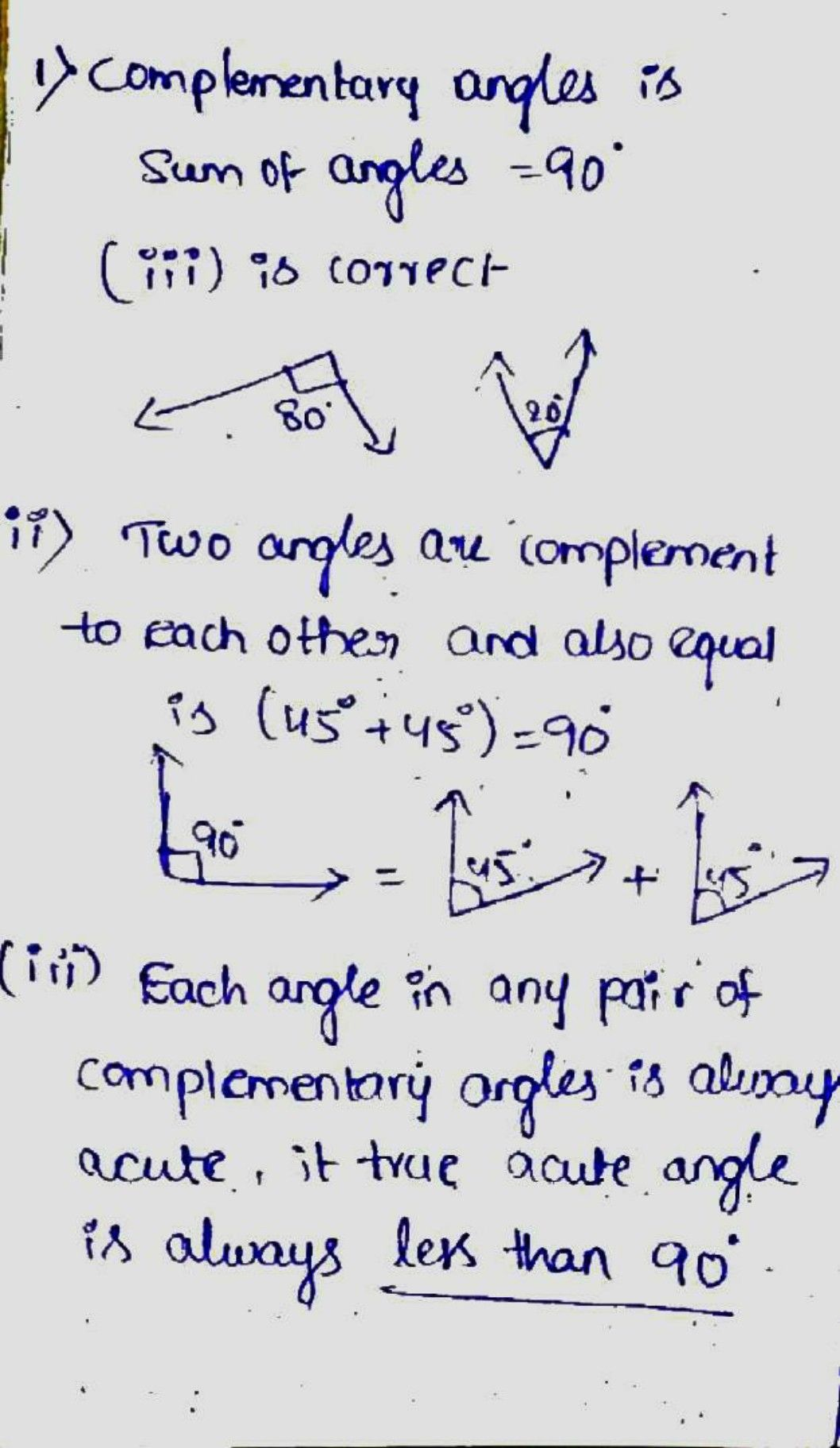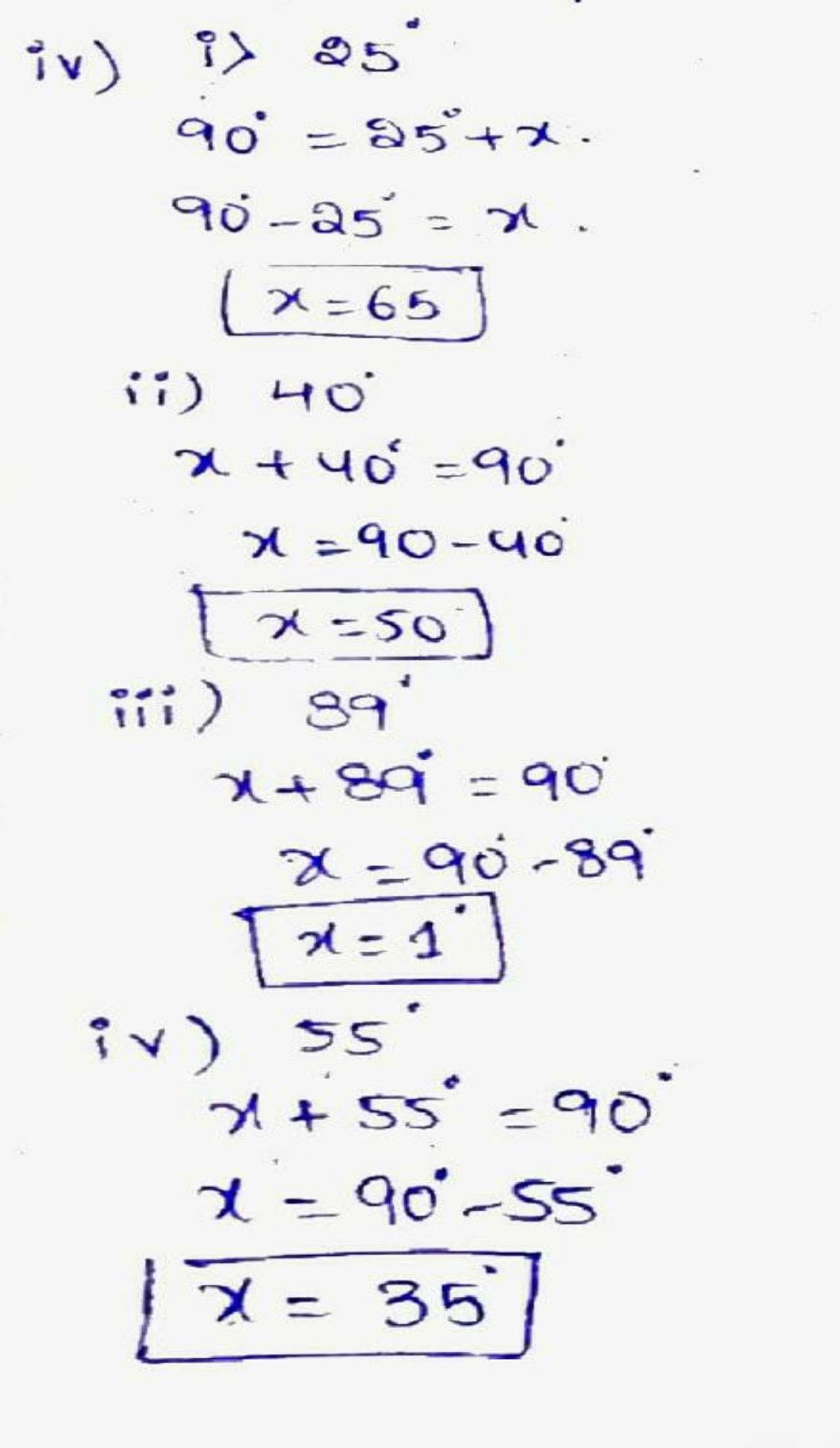Symbol
Problem1. Which of the following pairs of angles are complementary? $80^{2}$ $90^{°}$ $\sqrt{}$ $\bar{\sqrt{30^{°}} }$ $90^{°}$ $110^{°}$ $\left(1\right)$ $.$ $\left(\vec{1} 1\right)$ (111) $2.$ Find the complementary angles otf he following. $\left(1\right)$ $250$ $\left(1\vec{1} \right)$ $400$ $\left(\vec{1} \vec{1} 1\right)$ $890$ $\vec{1} \right)$ $550$ $3.$ Two angles are complement to each other and are also equal. Find them. 4. Manasa says, "Each angle in any pair of complementary angles is always acute". Do you agree? Give reason.
7th-9th grade
Geometry
Search count: 120
SolutionQanda teacher - NehaMamIf you have any doubt feel free to ask:) Dear, Please evaluate the answer and give gift coins(it does not reduce your coin balance.) for giving gift coins Click on evaluate. Then press the + icon 3 times(3000).
Please evaluate:- 1.Click on top right corner evaluate button. 2.Select 5 star 3.Click on gift coins + three times.(C3000)(not reduce your coins) 4. Click on DONE button on top right corner.Student
hi
mamQanda teacher - NehaMam
Please evaluate:- 1.Click on top right corner evaluate button. 2.Select 5 star 3.Click on gift coins + three times.(C3000)(not reduce your coins) 4. Click on DONE button on top right corner.